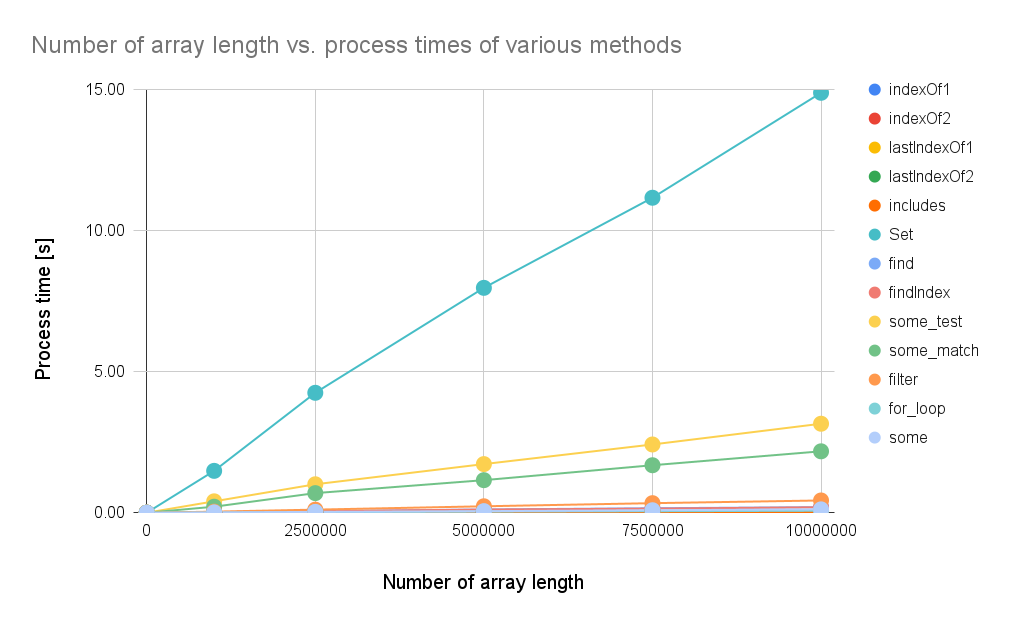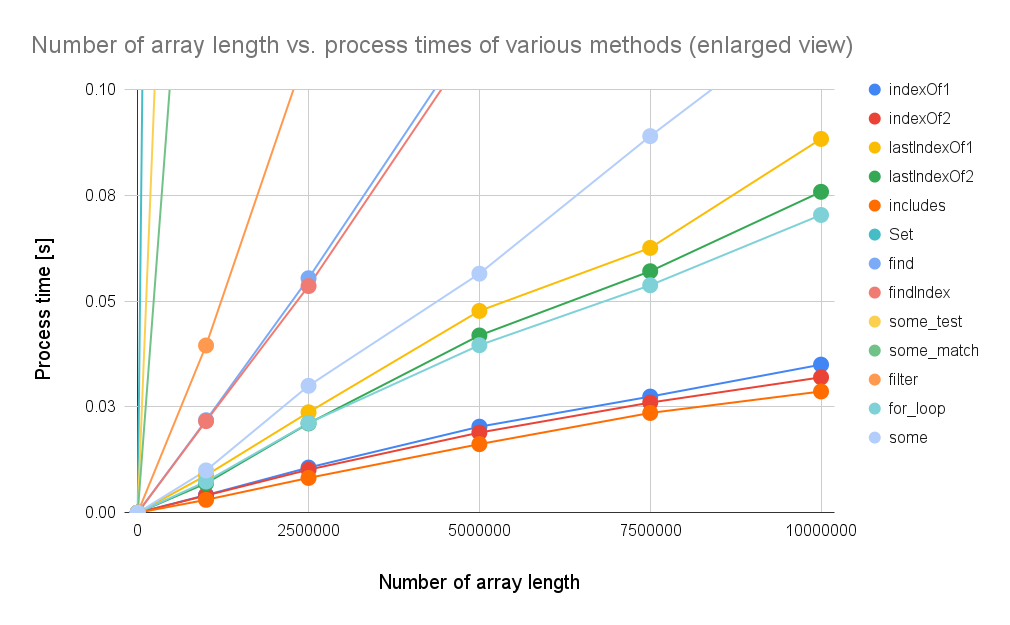# Benchmark: Process Costs for Checking Value in Array using Google Apps Script

Gists

Kanshi Tanaike

## Introduction

There is a maximum executing time for Google Apps Script (GAS). That is 6 minutes. And, in the case of the custom function and the simple trigger, it is 30 seconds. Ref So users always have to pay attention to reducing the process cost of the scripts. Especially, it is very important to know the process costs for the array processing, because array processing is often used for spreadsheets and Google APIs. I have already reported about the array processing at “Benchmark: Loop for Array Processing using Google Apps Script with V8” and “Search for Array Processing using Google Apps Script”. In this report, the process cost checking a value in a one-dimensional array using Google Apps Script has been investigated.

## Experimental procedure

In the experiment, a 1-dimensional array including a specific value in the center of the array was used. For example, if the array length is 11, the search value is put into the 6th element. The specific value is searched from the array using Google Apps Script under enabling V8 runtime. When the value is found, true is returned. This process was carried out by 13 methods, and the process costs (the processing time) were measured by changing the array length. You can see the sample scripts for these methods in “Appendix” section.

By the way, at GAS, the processing time is not stable as you know. So the average value for more than 200 times measurements was used for each data point which is shown by the figures. At this time, the fluctuation of the average values was less than 1 %. I worry that each detailed-data point in my environment might be different from that in another user’s environment. But I think that the trend of this result can be used.

## Results and discussionsFig. 1: Process times with increasing the number of array lengths.Fig. 2: Process times with increasing the number of array lengths. This figure is the enlarged view of Fig. 1.

Figure 1 shows the process times with increasing the number of array lengths. Figure 2 shows the enlarged view of Fig. 1.

From Figs. 1 and 2, the following results were obtained.

• The descending order of process costs is as follows. It was found that when a value is searched from the 1-dimensional array, the process cost of “includes” is the lowest of all.

Ascending of process costs Methods
1 includes
2 indexOf2
3 indexOf1
4 for loop
5 lastIndexOf2
6 lastIndexOf1
7 some
8 findIndex
9 find
10 filter
11 some_match
12 some_test
13 Set
• The process costs of “some_test”, “some_match” are higher than that of “some”. It is considered that the reason for this is due to the use of regex. When the regex is used, the process cost becomes high. And also, it was found that the process cost of “match” is lower than that of “test”.

• The process costs of “find” and “findIndex” are almost the same.

• When the value is compared with the equal, the process cost of “some” is lower than that of “find”.

• From the comparison of “lastIndexOf1” and “lastIndexOf2” are the comparison of “indexOf1” and “indexOf2”, the process cost of ~ of bitwise is lower than that of the comparison with = of equal. When ~ is used for comparing, the process costs of about 6 % and 12 % are reduced for “indexOf” and “lastIndexOf” with =, respectively.

• The process cost of “for loop” is lower than that of “lastIndexOf”. This is a very interesting result.

• About the benchmark of the loop process, you can see it at here.

## Summary

In this report, the process cost for checking a value in a 1-dimensional array using Google Apps Script was investigated. As the result, the following results were obtained.

• When a value is searched from the 1-dimensional array, the process cost of “includes” is the lowest of all.

• When it is checked whether a value is existing using a regex, the process cost of “match” is lower than that of “test”.

• When both “some” and “find” can be used, using “some” can reduce the process cost more than “find”.

• When the result is required to be retrieved as the boolean value, using ~ can reduce the process cost more than =.

As a note, I have to describe that this is the result for Google Apps Script. For other languages, this result might be different. And also, the process cost of this report might be modified by a future update of Google.

## Appendix

The following scripts were used for measuring the process cost.

const functions = [
{ name: "indexOf1", f: (array => array.indexOf(search) != -1) },
{ name: "indexOf2", f: (array => Boolean(~array.indexOf(search))) },
{ name: "lastIndexOf1", f: (array => array.lastIndexOf(search) != -1) },
{ name: "lastIndexOf2", f: (array => Boolean(~array.lastIndexOf(search))) },
{ name: "includes", f: (array => array.includes(search)) },
{ name: "Set", f: (array => new Set(array).has(search)) },
{ name: "find", f: (array => Boolean(array.find(e => e == search))) },
{ name: "findIndex", f: (array => Boolean(array.findIndex(e => e == search))) },
{ name: "some_test", f: (array => array.some(e => new RegExp(search).test(e))) },
{ name: "some_match", f: (array => array.some(e => e.match(new RegExp(search)))) },
{ name: "some", f: (array => array.some(e => e == search)) },
{ name: "filter", f: (array => Boolean(array.filter(e => e == search).length)) },
{
name: "for_loop", f: (array => {
let res = false;
for (let i = 0; i < array.length; i++) {
if (array[i] == search) {
res = true;
break;
}
}
return res;
})
},
];